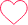# Decimal To Octal Conversion | C Program

## Decimal to Octal Conversion in C

Here, in this section, we will discuss the Decimal to Octal conversion in C.

The C program to convert decimal to octal number accepts a decimal number from the user. This number is further converted to its equivalent octal number after following a series of steps. The following section gives an algorithm for this conversion. It is then followed by a C program.## Methods discussed

• Using an array to store the comparable octal value
• Using int variable to store comparable octal value

### Method 1## C Program for Decimal to Octal Conversion

Run
```// C Program to convert Decimal to Octal using Array
#include<stdio.h>

void convert(int num)
{
// creating an array to store octal equivalent
int octalArray;

// using i to store octal bit at given array position
int i = 0;
while (num > 0) {

// resultant remainder is stored at given array position
octalArray[i] = num % 8;
num = num / 8;
i++;
}

// printing octal array in reverse order
for (int j = i - 1; j >= 0; j--)
printf("%d",octalArray[j]);
}

int main()
{
int n = 148;
convert(n);
return 0;
}```

`224`

## Method 2

• Rather than creating a temporary array
• We will use a variable decimal to store the octal result
• Will use a mathematical calculation to derive conversion into variable### Method 2 Code in C

Run
```#include<stdio.h>

void convert(int num)
{
int octal = 0;
int rem, i = 1;

while(num!=0)
{
rem = num % 8;
num /= 8;
octal += rem * i;

// moving to next position ex: units -> tens
i *= 10;
}
printf("%d",octal);
}

int main()
{
int decimal_num = 221;
convert(decimal_num);
return 0;
}```

`335`

### Related Banners

Get PrepInsta Prime & get Access to all 200+ courses offered by PrepInsta in One Subscription

### 2 comments on “Decimal To Octal Conversion | C Program”

•J

#include

int main()
{
int n,decimal,rem,sum=0,base=1;
printf(“enter a decimal number\n”);
scanf(“%d”,&decimal);
n=decimal;
while(decimal>0)
{
rem=decimal%8;
sum=sum+rem*base;
decimal=decimal/8;
base=base*10;
}
printf(“the octal format is %d “,sum);
}
simplest form for above progam9
•HelpPrepInsta

Thanks for contributing the code Madhavi1